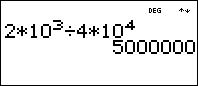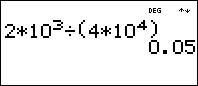# Knowledge Base

## Solution 20469: Incorrect Answer Received When Dividing Two Values in Scientific Notation Using the TI-30XS/B MultiView™ and TI-34 MultiView™ Scientific Calculators.

### Why do I receive an incorrect answer when I divide two values in scientific notation using the TI-30XS/B MultiView and TI-34 MultiView?

The TI-30XS/B MultiView and TI-34 MultiView calculators have a specific scientific notation shortcut key unique to all other scientific calculators, [x10n].

When dividing two values in scientific notation, it is necessary to put parentheses around the second value in order to keep the expression from being calculated using the standard order of operations. If parentheses are omitted, the calculator will yield an incorrect result. The examples below illustrate the incorrect and correct input when diving two values in scientific notation using the TI-30XS MultiVew and TI-34 MultiView:

INCORRECT INPUT                       CORRECT INPUTPlease see the TI-30XS/B MultiView and TI-34 MultiView guidebooks for additional information.